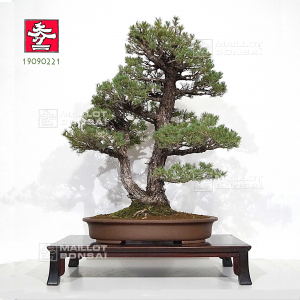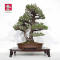##### The Japanese Bonsai specialist
Direct order Contact Help / Services Newsletter# Pin 5 aiguilles kokonoe ref 19090221

Pinus pentaphylla "kokonoe"ref. : 11779

2.530,00

voluminous/heavy item extra shipping of 12,00

Available quantity : 1Order

###### Description

Japanese white pine, Kokonoe variety.

Height of the tree only: 760 mm.

Width of the branches: 710 mm.

Dimensions of the quality non-enameled pot: 560*440*95 mm.

Diameter of the trunks: 45 and 65 mm.

Nebari: 150*170 mm. No strong cutting wound or wire mark, superb ramifications: the true 2-trunk sokan style. This bonsaï is more than 40/45 years old with a perfectly formed bark and good cohesion between the 2 trunks. For collectors.

The kokonoe variety with short needles has the particularity to re-bud easily in the backside, which makes it easier to form beautiful and dense trays.

Shelf not included. Photographed in September 2022.

#kokonoe 4.7 #bonsai 3.6 #masterpieces 3.2 #aiguilles 2.9 #variety 2.7 #trunks 2.6 #with 2.4 #ramifications 2.3 #particularity 2.3 #photographed 2.2

Formule
(( ROUND((CHAR_LENGTH(b.article_nom)-CHAR_LENGTH(REPLACE(b.article_nom, 'kokonoe', '')))/LENGTH('kokonoe')) + ROUND((CHAR_LENGTH(b.article_description)-CHAR_LENGTH(REPLACE(b.article_description, 'kokonoe', '')))/LENGTH('kokonoe')) ) * 4.7) + (( ROUND((CHAR_LENGTH(b.article_nom)-CHAR_LENGTH(REPLACE(b.article_nom, 'aiguilles', '')))/LENGTH('aiguilles')) + ROUND((CHAR_LENGTH(b.article_description)-CHAR_LENGTH(REPLACE(b.article_description, 'aiguilles', '')))/LENGTH('aiguilles')) ) * 2.9) + (( ROUND((CHAR_LENGTH(b.article_nom)-CHAR_LENGTH(REPLACE(b.article_nom, 'variety', '')))/LENGTH('variety')) + ROUND((CHAR_LENGTH(b.article_description)-CHAR_LENGTH(REPLACE(b.article_description, 'variety', '')))/LENGTH('variety')) ) * 2.7) + (( ROUND((CHAR_LENGTH(b.article_nom)-CHAR_LENGTH(REPLACE(b.article_nom, 'trunks', '')))/LENGTH('trunks')) + ROUND((CHAR_LENGTH(b.article_description)-CHAR_LENGTH(REPLACE(b.article_description, 'trunks', '')))/LENGTH('trunks')) ) * 2.6) + (( ROUND((CHAR_LENGTH(b.article_nom)-CHAR_LENGTH(REPLACE(b.article_nom, 'with', '')))/LENGTH('with')) + ROUND((CHAR_LENGTH(b.article_description)-CHAR_LENGTH(REPLACE(b.article_description, 'with', '')))/LENGTH('with')) ) * 2.4) + (( ROUND((CHAR_LENGTH(b.article_nom)-CHAR_LENGTH(REPLACE(b.article_nom, 'particularity', '')))/LENGTH('particularity')) + ROUND((CHAR_LENGTH(b.article_description)-CHAR_LENGTH(REPLACE(b.article_description, 'particularity', '')))/LENGTH('particularity')) ) * 2.3) + (( ROUND((CHAR_LENGTH(b.article_nom)-CHAR_LENGTH(REPLACE(b.article_nom, 'ramifications', '')))/LENGTH('ramifications')) + ROUND((CHAR_LENGTH(b.article_description)-CHAR_LENGTH(REPLACE(b.article_description, 'ramifications', '')))/LENGTH('ramifications')) ) * 2.3) + (( ROUND((CHAR_LENGTH(b.article_nom)-CHAR_LENGTH(REPLACE(b.article_nom, 'photographed', '')))/LENGTH('photographed')) + ROUND((CHAR_LENGTH(b.article_description)-CHAR_LENGTH(REPLACE(b.article_description, 'photographed', '')))/LENGTH('photographed')) ) * 2.2) + (( ROUND((CHAR_LENGTH(b.article_nom)-CHAR_LENGTH(REPLACE(b.article_nom, 'dimensions', '')))/LENGTH('dimensions')) + ROUND((CHAR_LENGTH(b.article_description)-CHAR_LENGTH(REPLACE(b.article_description, 'dimensions', '')))/LENGTH('dimensions')) ) * 2) + (( ROUND((CHAR_LENGTH(b.article_nom)-CHAR_LENGTH(REPLACE(b.article_nom, 'collectors', '')))/LENGTH('collectors')) + ROUND((CHAR_LENGTH(b.article_description)-CHAR_LENGTH(REPLACE(b.article_description, 'collectors', '')))/LENGTH('collectors')) ) * 2)

## Secure payment## Delivery

Our logistic partners :04 74 55 23 48
Pépinière MAILLOT-BONSAÏ
Le Bois Frazy
01990 RELEVANT - FRANCE
on appointment